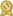## specializes in Mathematics and Statistics tutor · male

"I studied mathematics and physics at Arizona State University and have been tutoring mathematics since January 2013 (I tutored at the largest math tutoring center in the nation (ASU's) for 3 years and got a tutor of the year reward there one year). I am always ready to learn more math and share any knowledge of it with other people (in fact I enjoy doing both!). I believe the best way to learn math is to more...

"I studied mathematics and physics at Arizona State University and have been tutoring mathematics since January 2013 (I tutored at the largest math tutoring center in the nation (ASU's) for 3 years and got a tutor of the year reward there one year). I am always ready to learn more math and share any knowledge of it with other people (in fact I enjoy doing both!). I believe the best way to learn math is to understand the underlying concepts behind mathematical problems, to understand the problem-solving techniques used to solve specific 'types' of problems, and to question why (to be curious about) certain mathematical truths are what they are.

In High School, I took (Geometry and Algebra) Calculus I and Calculus II and received a 5 on the AP Calculus B/C exam. At ASU, I have taken (for math or math-related) Math for Business Analysis (MAT211), Introduction to Programming in Java (CSE110), Calculus III (MAT272), Differential Equations (MAT275), Linear Algebra (MAT342), Mathematical Structures (MAT300), Discrete Mathematics (MAT243), Advanced Calculus I (MAT371), Introduction to Abstract Algebra (MAT443), Cryptography I (MAT447), Number Theory (MAT445), Cryptography II (MAT448), Intermediate Abstract Algebra (MAT444), IEE380 (Stats and Probability), Differential Geometry (MAT494), Honors Microeconomics (ECN214), Economics of Money and Banking (ECN315), Probability (STP421), Physics I, II, & III (PHY150,151,252), and Mathematical Methods for Physics II (PHY302), General Relativity (PHY523), and Classical & Continuum Mechanics (PHY521).

I'm a CRLA Certified Tutor." less...

# Details

 fee: \$45 (for 60 min) travel distance: 10 miles meeting type: online (only)

# Contact

 website: on file

# Education and Qualifications

 certified:Arizona State University, Mathematics

# Subjects Tutored

Algebra 1 - Algebra 2 - Calculus - Microsoft Excel - Physics - Precalculus - Trigonometry - Statistics - Discrete Math - Differential Equations - Linear Algebra